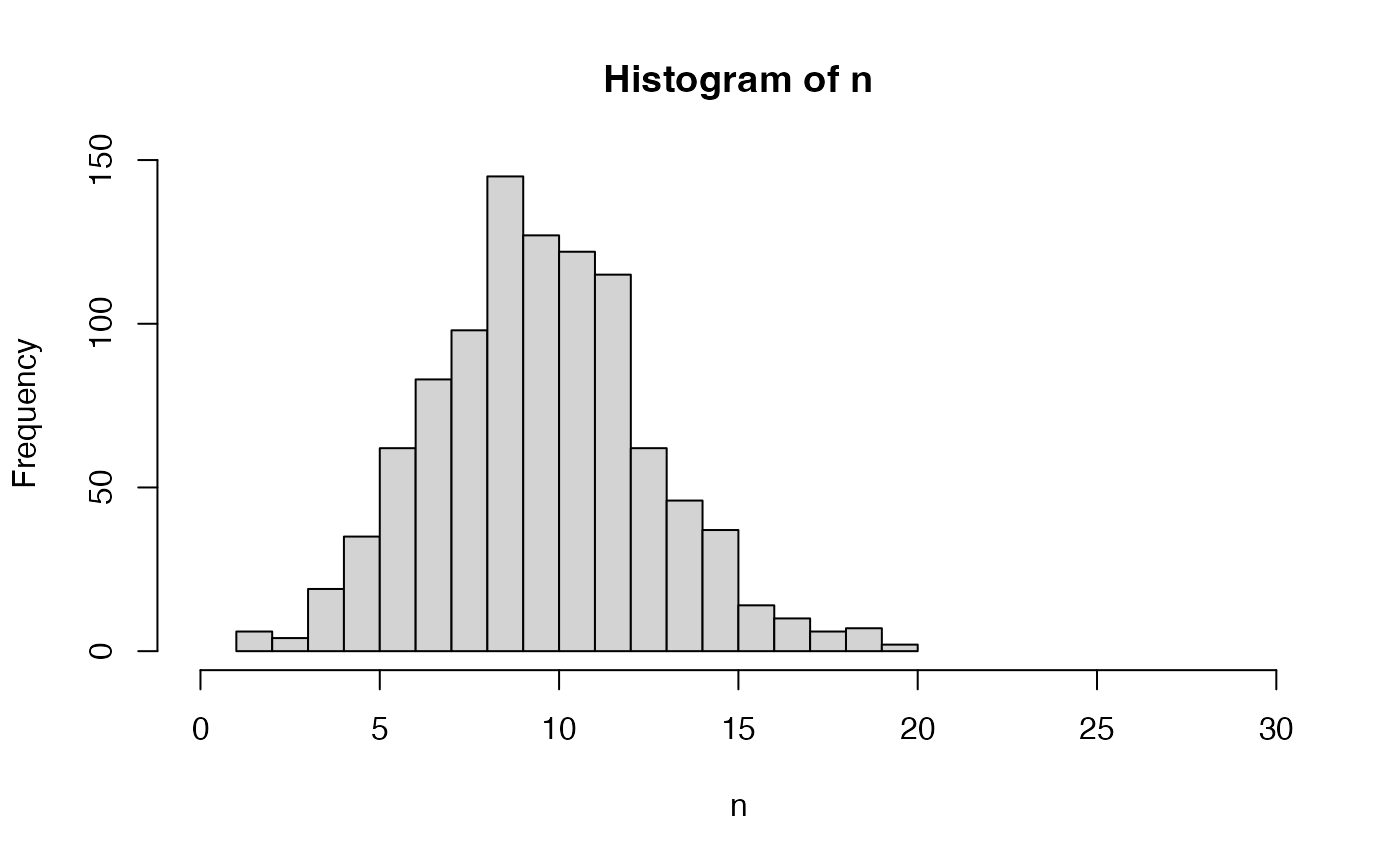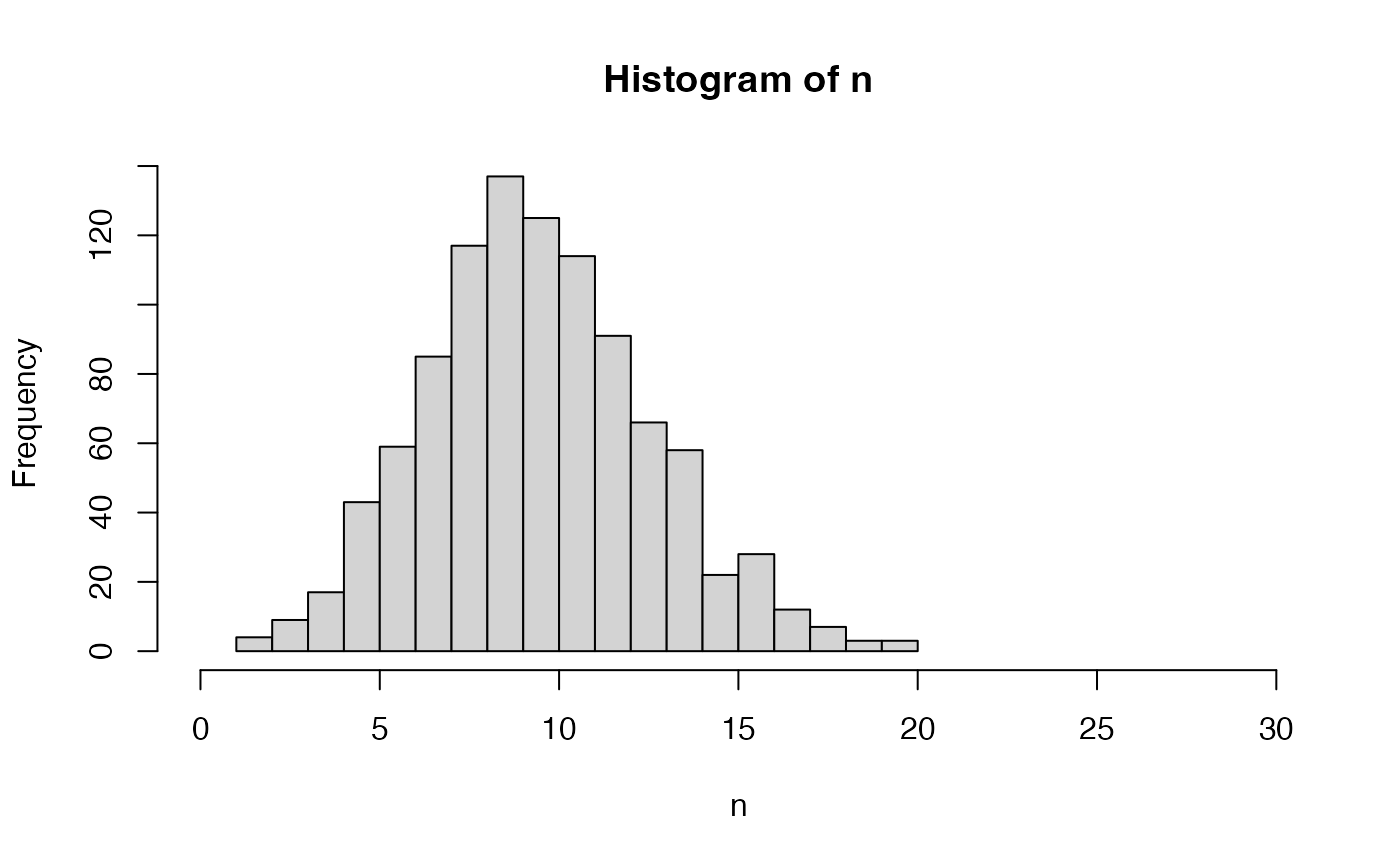Sample a number of virgin queens - used when nFathers = NULL (see SimParamBee$nVirginQueens). This is just an example. You can provide your own functions that satisfy your needs! nVirginQueensPoisson(colony, n = 1, average = 10) nVirginQueensTruncPoisson(colony, n = 1, average = 10, lowerLimit = 0) nVirginQueensColonyPhenotype( colony, queenTrait = 1, workersTrait = 2, checkProduction = FALSE, lowerLimit = 0, ... ) ## Arguments colony Colony-class n integer, number of samples average numeric, average number of virgin queens lowerLimit numeric, returned numbers will be above this value queenTrait numeric (column position) or character (column name), trait that represents queen's effect on the colony phenotype (defined in SimParamBee - see examples); if NULL then this effect is 0 workersTrait numeric (column position) or character (column name), trait that represents workers's effect on the colony phenotype (defined in SimParamBee - see examples); if NULL then this effect is 0 checkProduction logical, does the phenotype depend on the production status of colony; if yes and production is not TRUE, the result is above lowerLimit ... other arguments of mapCasteToColonyPheno ## Value numeric, number of virgin queens ## Details nVirginQueensPoisson samples from a Poisson distribution, which can return a value 0 (that would mean a colony will fail to raise a single virgin queen after the queen swarms or dies). nVirginQueensTruncPoisson samples from a truncated Poisson distribution (truncated at zero) to avoid failure. nVirginQueensColonyPhenotype returns a number (above lowerLimit) as a function of colony phenotype, say swarming tendency. Colony phenotype is provided by mapCasteToColonyPheno. You need to set up traits influencing the colony phenotype and their parameters (mean and variances) via SimParamBee (see examples). ## Functions • nVirginQueensTruncPoisson(): Sample a non-zero number of virgin queens • nVirginQueensColonyPhenotype(): Sample a non-zero number of virgin queens based on colony's phenotype, say, swarming tendency ## See also SimParamBee field nVirginQueens and vignette(topic = "QuantitativeGenetics", package = "SIMplyBee") ## Examples nVirginQueensPoisson() #>  9 nVirginQueensPoisson() #>  10 n <- nVirginQueensPoisson(n = 1000) hist(n, breaks = seq(from = min(n), to = max(n)), xlim = c(0, 30))table(n) #> n #> 2 3 4 5 6 7 8 9 10 11 12 13 14 15 16 17 18 19 20 #> 5 9 24 36 68 82 113 124 138 122 84 69 55 30 19 11 6 4 1 nVirginQueensTruncPoisson() #>  7 nVirginQueensTruncPoisson() #>  11 n <- nVirginQueensTruncPoisson(n = 1000) hist(n, breaks = seq(from = min(n), to = max(n)), xlim = c(0, 30))table(n) #> n #> 1 2 3 4 5 6 7 8 9 10 11 12 13 14 15 16 17 18 20 21 #> 1 1 9 14 45 64 81 127 112 148 106 110 56 51 31 20 12 9 2 1 # Example for nVirginQueensColonyPhenotype() founderGenomes <- quickHaplo(nInd = 3, nChr = 1, segSites = 100) SP <- SimParamBee$new(founderGenomes)
# Setting trait scale such that mean is 10 split into queen and workers effects
meanP <- c(5, 5 / SP$nWorkers) # setup variances such that the total phenotype variance will match the mean varA <- c(3 / 2, 3 / 2 / SP$nWorkers)
corA <- matrix(data = c(
1.0, -0.5,
-0.5, 1.0
), nrow = 2, byrow = TRUE)
varE <- c(7 / 2, 7 / 2 / SP$nWorkers) varA / (varA + varE) #>  0.3 0.3 varP <- varA + varE varP + varP * SP$nWorkers
#>  10
SP$addTraitA(nQtlPerChr = 100, mean = meanP, var = varA, corA = corA) SP$setVarE(varE = varE)
basePop <- createVirginQueens(founderGenomes)
#> Error in get(x = "SP", envir = .GlobalEnv): object 'SP' not found
drones <- createDrones(x = basePop, nInd = 50)
#> Error in get(x = "SP", envir = .GlobalEnv): object 'SP' not found
droneGroups <- pullDroneGroupsFromDCA(drones, n = 2, nDrones = 15)
#> Error in get(x = "SP", envir = .GlobalEnv): object 'SP' not found
colony1 <- createColony(x = basePop)
#> Error in get(x = "SP", envir = .GlobalEnv): object 'SP' not found
colony2 <- createColony(x = basePop)
#> Error in get(x = "SP", envir = .GlobalEnv): object 'SP' not found
colony1 <- cross(colony1, drones = droneGroups[])
#> Error in get(x = "SP", envir = .GlobalEnv): object 'SP' not found
colony2 <- cross(colony2, drones = droneGroups[])
#> Error in get(x = "SP", envir = .GlobalEnv): object 'SP' not found
colony1 <- buildUp(colony1)
#> Error in get(x = "SP", envir = .GlobalEnv): object 'SP' not found
colony2 <- buildUp(colony2)
#> Error in get(x = "SP", envir = .GlobalEnv): object 'SP' not found
nVirginQueensColonyPhenotype(colony1)
#> Error in get(x = "SP", envir = .GlobalEnv): object 'SP' not found
nVirginQueensColonyPhenotype(colony2)
#> Error in get(x = "SP", envir = .GlobalEnv): object 'SP' not found